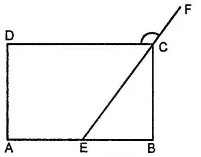(a) What is the minimum interior angle possible for a regular polygon

(b) What is the maximum exterior angle possible for a regular polygon

Asked by Aaryan | 1 year ago |  85

##### Solution :-

Consider a regular polygon having the lowest possible number of sides (i.e., an equilateral triangle). The exterior angle of this triangle will be the maximum exterior angle possible for any regular polygon.

Exterior angle of an equilateral triangle $$\cfrac{360^\circ}{3} = 120^\circ$$

Hence, maximum possible measure of exterior angle for any polygon is 120º. Also, we know that an exterior angle and an interior angle are always in a linear pair.

Hence, minimum interior angle = 180º - 120° = 60º

Answered by Sakshi | 1 year ago

### Related Questions

#### In the given figure, PQRS is a kite. Find the values of x and y.

In the given figure, PQRS is a kite. Find the values of x and y.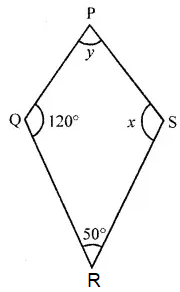#### In the given isosceles trapezium ABCD, ∠C = 102°. Find all the remaining angles of the trapezium.

In the given isosceles trapezium ABCD, ∠C = 102°. Find all the remaining angles of the trapezium.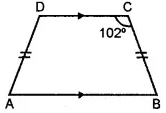#### In the given figure, ABCD is a rhombus and ∠ABD = 50°. Find :

In the given figure, ABCD is a rhombus and ∠ABD = 50°. Find :

(i) ∠CAB

(ii) ∠BCD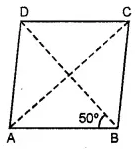#### In the given figure, ABCD is a rectangle and diagonals intersect at O. If ∠AOB = 118°, find

In the given figure, ABCD is a rectangle and diagonals intersect at O. If ∠AOB = 118°, find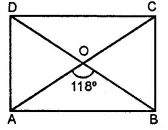(i) ∠ABO

(iii) ∠OCB